## Introduction

Consider the vertical lines given below. How do we find out how many vertical lines there are?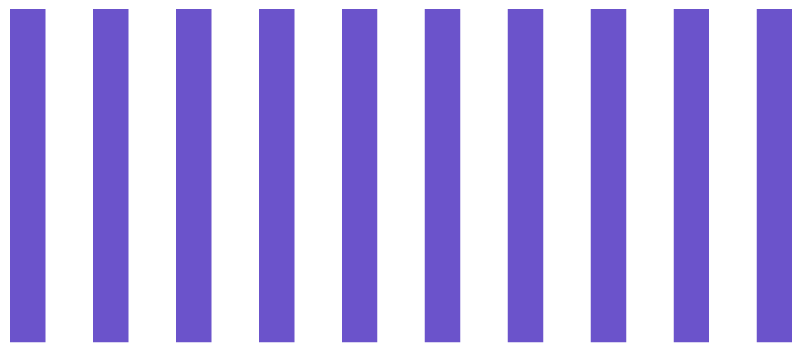The answer is by counting. We say that there are 10 vertical lines. Here we start counting from 1 and then 2, 3, 4, …etc. There can be different ways of counting by counting in 1s or in groups. Based on the concept of counting, we find that the counting number starts from 1 and has infinitely many numbers. These counting numbers are called natural numbers.

‘0’ and the set of natural numbers form a new set of numbers called whole numbers. So, we can see that the need of zero has given rise to a new number system called whole numbers.

Watch this video below to know the different types of number systems and their need:

The collection of negative numbers..., – 4, – 3, – 2, – 1, 0, 1, 2, 3, 4, ... is called integers.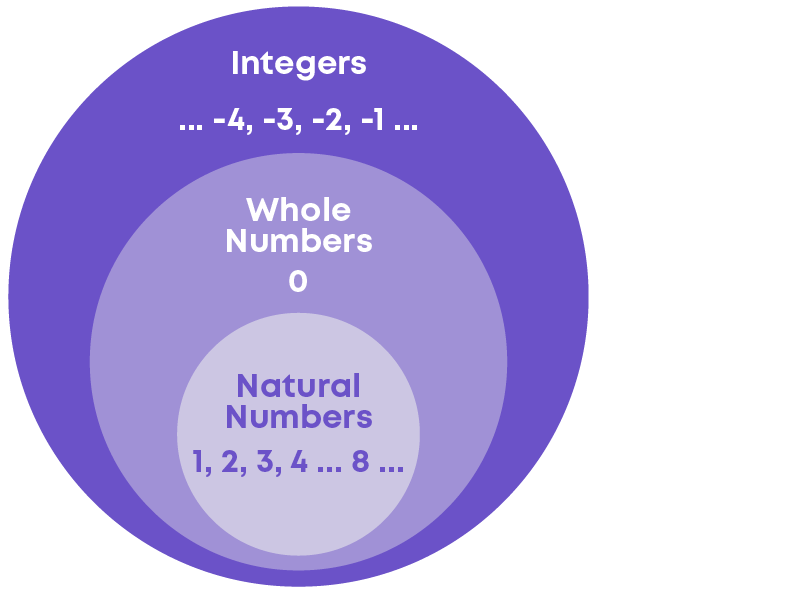What are fractions? Fractions are the numbers of the form p q where p and q are whole numbers and q ≠ 0.

Numbers of the form p q where ‘p’ and ‘q’ are integers and q ≠ 0 are called rational numbers.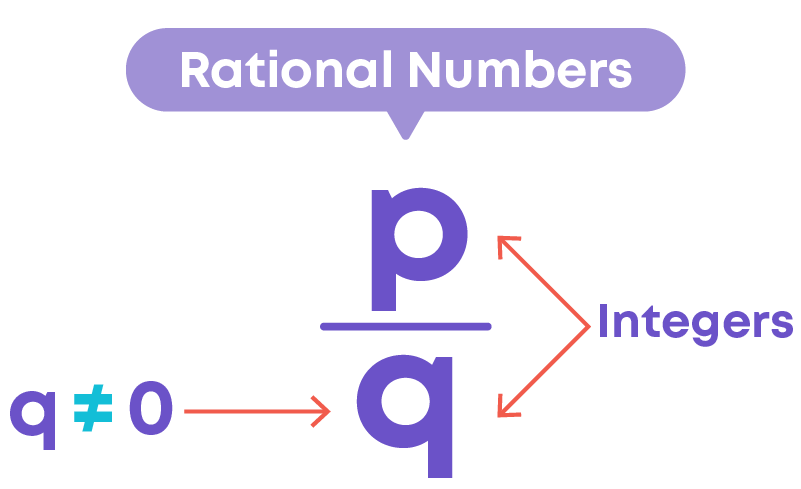Natural numbers, whole numbers, decimals, and integers can be expressed as rational numbers. Hence, we can conclude that all natural numbers, whole numbers, fractions, decimals, and integers are rational numbers.

If a number is not a rational, then it is not natural, whole or an integer. So, what do we call the numbers that are not rational? Numbers that are not rational numbers are called as irrational numbers. These are numbers that are not in the form of p q where ‘p’ and ‘q’ are

integers and q ≠ 0. Examples: √2, √3, etc.

All the above number systems put together gives rise to another number system called the real number system.Real numbers are numbers which are either rational or irrational. Examples: 4, -9, 6.34, 3 4 , √4, etc.

#### Concepts

The chapter ‘Number System’ covers the following concepts:

#### Real Numbers and Their Decimal Expansions

A number ‘s’ is called irrational if it cannot be written in the form p q where p and q are integers and q ≠ 0. Examples: √2, √3, etc.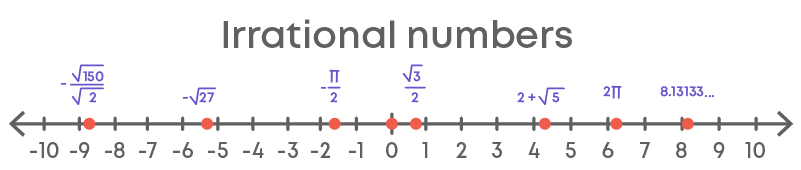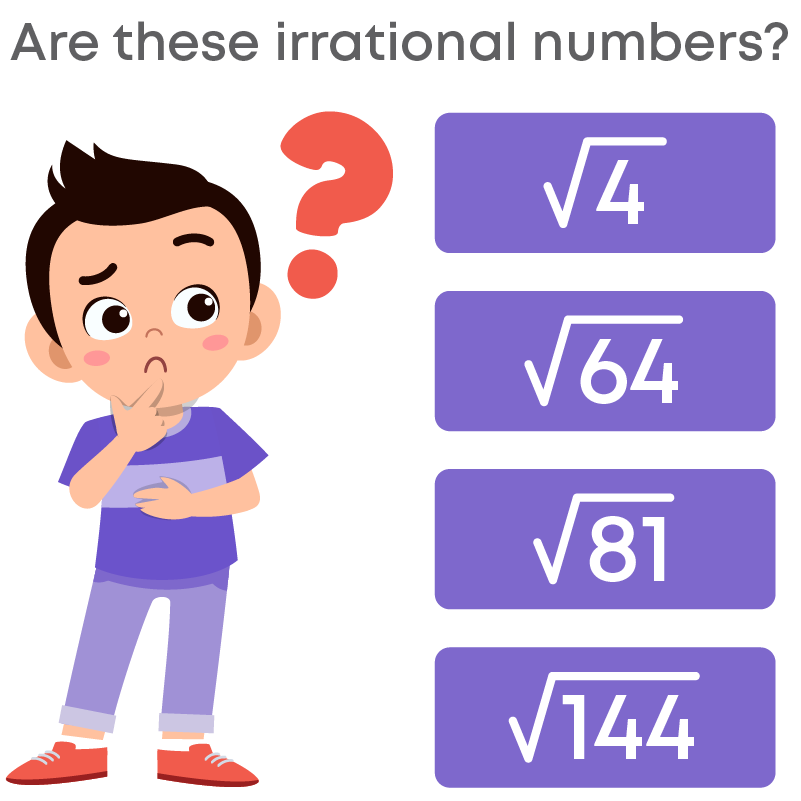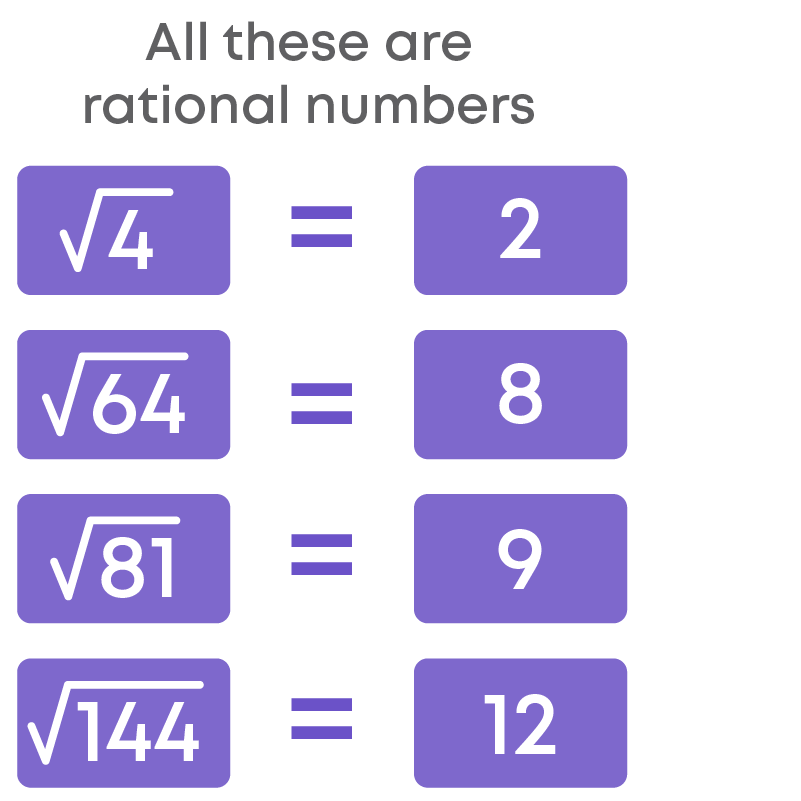So, we can conclude that not all numbers under the square root are irrational numbers.

Consider the irrationals √3 and √5. Their sum = √3 + √5 cannot be simplified further. Also, the result is irrational.

Consider the irrational numbers 3√2 and 2√2. ​Here, we can see that these numbers have the same irrational number √2. Let us add them.

Here, the irrational numbers have the same irrational coefficient. Hence, we can add the rational coefficients.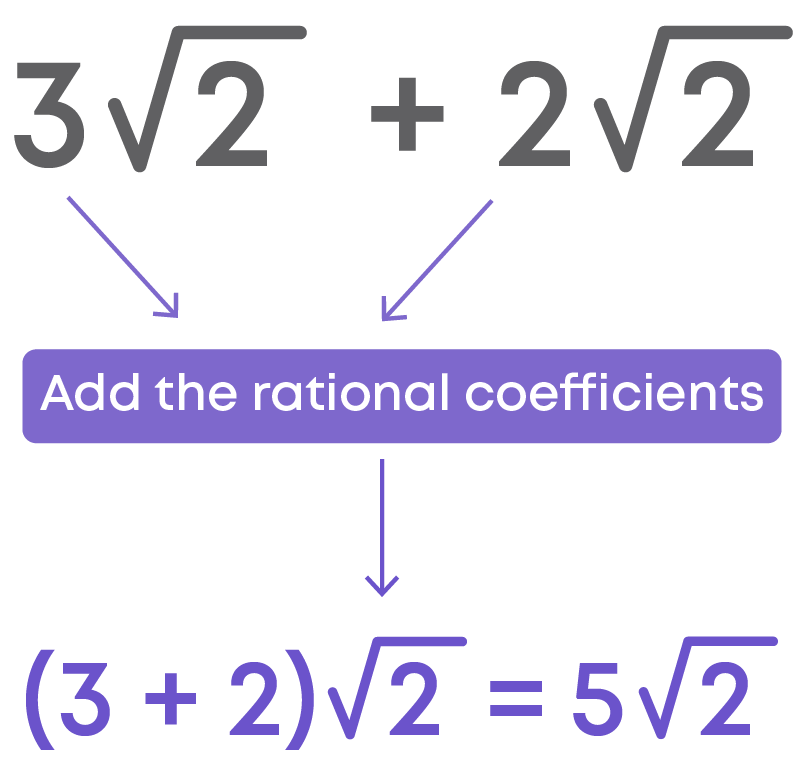The result is an irrational number. The sum of two irrational numbers can be an irrational number.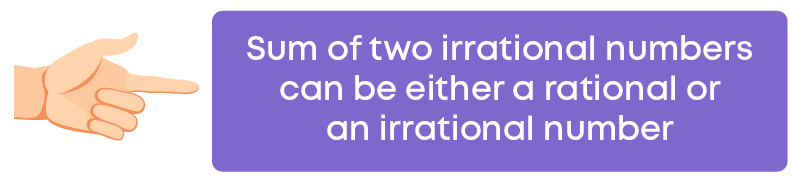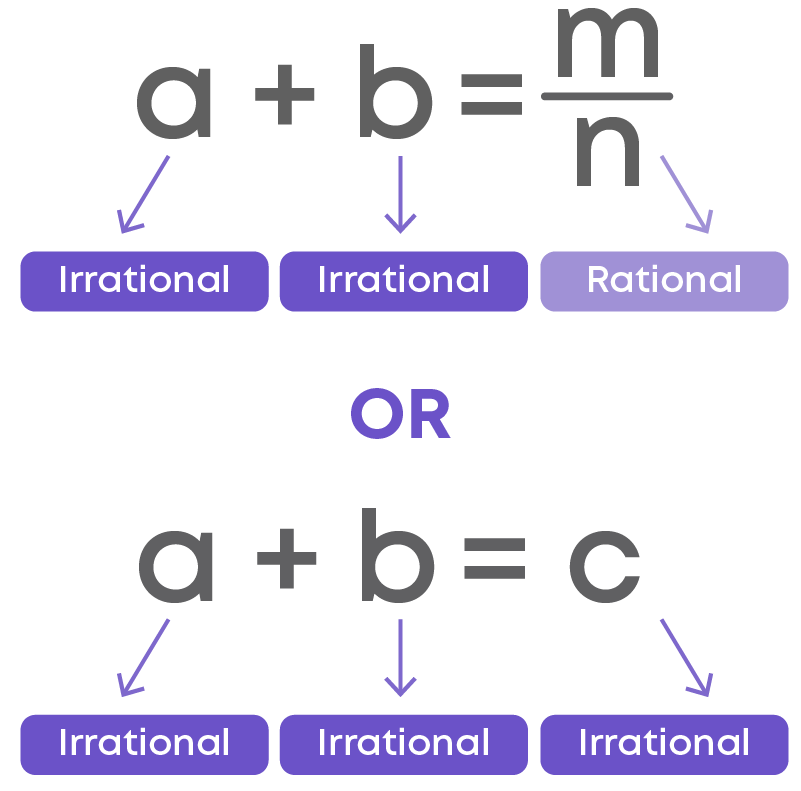Let us consider two irrational numbers, say √2 and √3. Their difference is √2 - √3 which is an irrational number.

The difference between two irrational numbers may be rational or irrational.

The difference between irrational numbers that are rational are √3 – √3, √9 – √49, etc.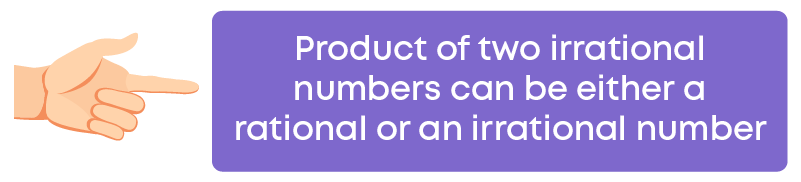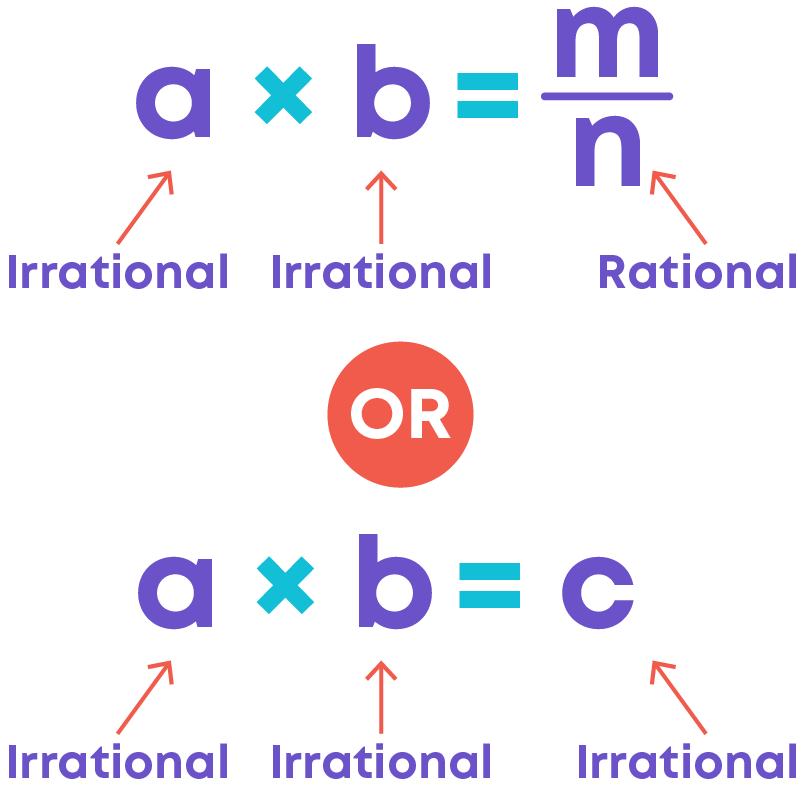Examples: √3 × √3 = √32 = 3, where 3 is a rational number, and

√2 × √5 = √10, where √10 is an irrational number.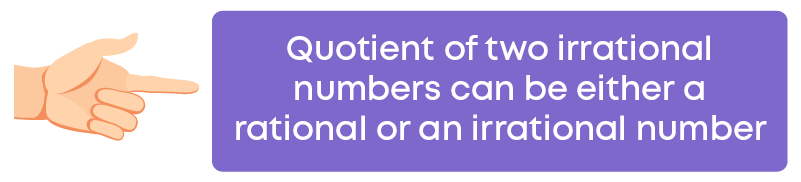Examples: √5 √5 = 1, where 1 is a rational number, and

√22 √11 = √2, where √2 is an irrational number.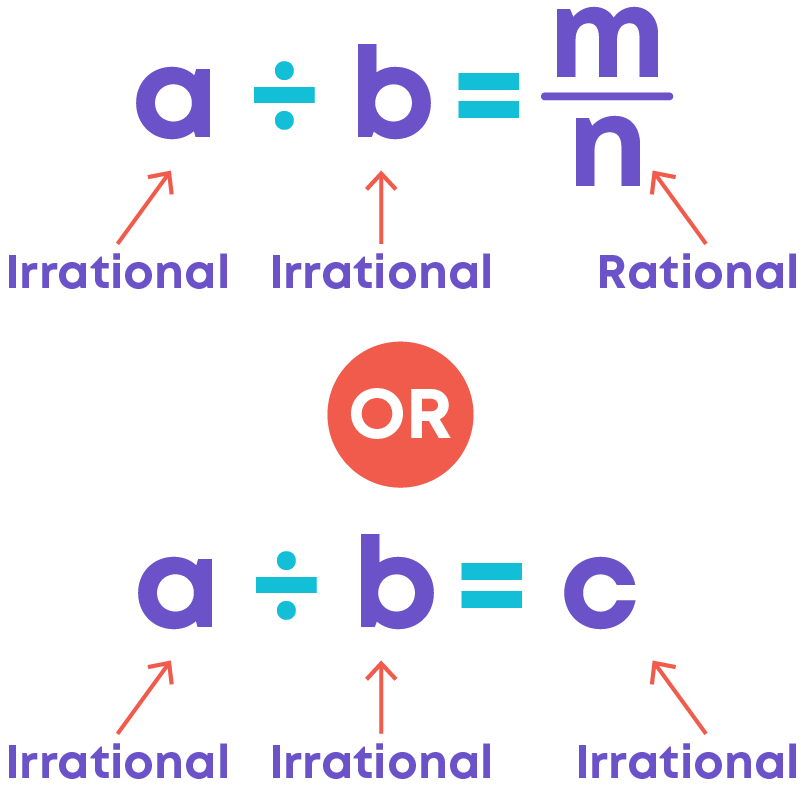To locate the irrational number of the form √n where ‘n’ is a positive integer on the number line, we need to follow the steps given below:

Step I – Write the given number (without root) as the sum of the squares of two numbers (say ‘a’ and ‘b’, where a > b) such that n = a2 + b2

Step II – Construct a right-angled triangle on the number line using the lengths ‘a’, ‘b’ and the hypotenuse as ‘n’

• Draw a real number line.
• From 0, mark a distance equal to ‘a’ on the number line.
• Mark this point as A.
• At A, construct a perpendicular to the number line.
• On this perpendicular, cut off a length ‘b’ unit. Mark this point as B.
• Join OB.
• Now, take a length of OB on the compass. With O as center, cut off the length OB on the number line at C.
• The point C represents the required irrational number.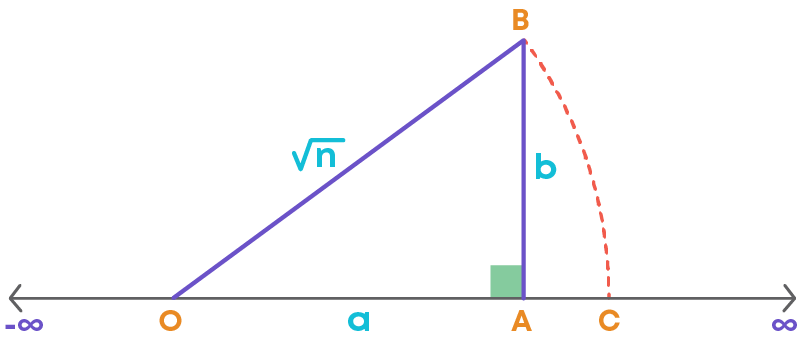Thus, point C will represent the location of √n on the number line.

Let us represent √2 on the number line.

Step I – Write the given number (without root) as the sum of the squares of two natural numbers (say ‘a’ and ‘b’, where a > b). The number without root is 2.

2 can be written as 2 = 12 + 12

Step II – Construct a right-angled triangle on the number line using the lengths ‘a’, ‘b’ and the hypotenuse as ‘n’.

• Draw a real number line.
• From 0, mark a distance equal to 1 on the number line.
• Mark this point as A.
• At A, construct a perpendicular to the number line.
• On this perpendicular, cut off a length 1 unit. Mark this point as B.
• Join OB.
• Now, take a length of OB on the compass. With O as center, cut off the length OB on the number line at C.
• The length OC represents the required irrational number.Let us consider the irrationals like √3, √6, etc. Can these numbers be written as sum of squares of two natural numbers? No.

How do we represent these irrational numbers as sum of squares of two numbers? We can use an irrational and a rational number, that is (√2)2 + 12 = 2 + 1 = 3 → √3

Let us represent √3 on the number line.

Step 1: Write the given number (without root) as the sum of the squares of two numbers,

that is, (√2)2 + 12

This means, that we:

• First, we must represent √2 on the number line.
• With this as base, we can construct another right-angled triangle with the perpendicular side 1 unit.
• The hypotenuse of this triangle will be the value of √3.

Step 2: Represent √2 on the number line

2 = 12 + 12

Construct a right-angled triangle on the number line using the lengths ‘1’, ‘1’ and the hypotenuse as √2

• Draw a real number line.
• From 0, mark a distance equal to 1 on the number line.
• Mark this point as A.
• At A, construct a perpendicular to the number line.
• On this perpendicular, cut off a length 1 unit. Mark this point as B.
• Join OB.
• Now, take a length of OB on the compass. With O as center, cut off the length OB on the number line at E.
• The length OE represents the irrational number √2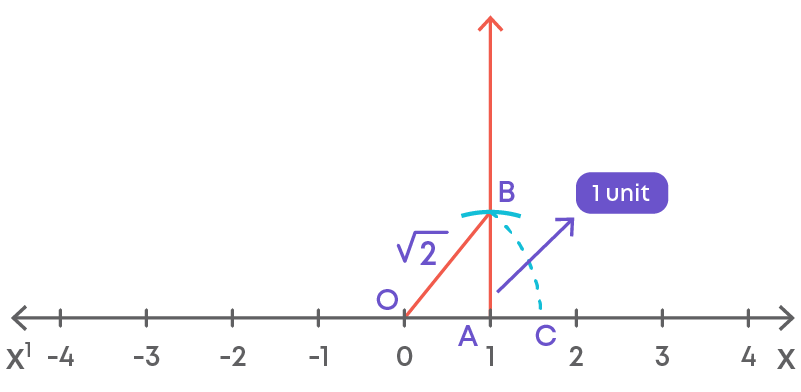Step 3: Represent √3 on the number line

Now to represent √3 on the number line, we need to repeat the steps followed to represent √2.

The triangle should be constructed using the length OB as the base.

• Draw a perpendicular line BC at B which will measure 1 unit.
• Join the O and point C.
• Now, take a length of OC on the compass. With O as center, cut off the length OC on the number line at F.
• The point F represents the irrational number √3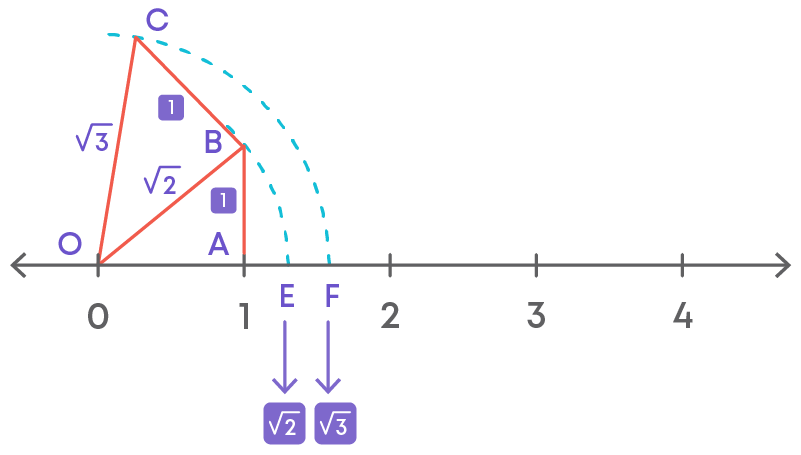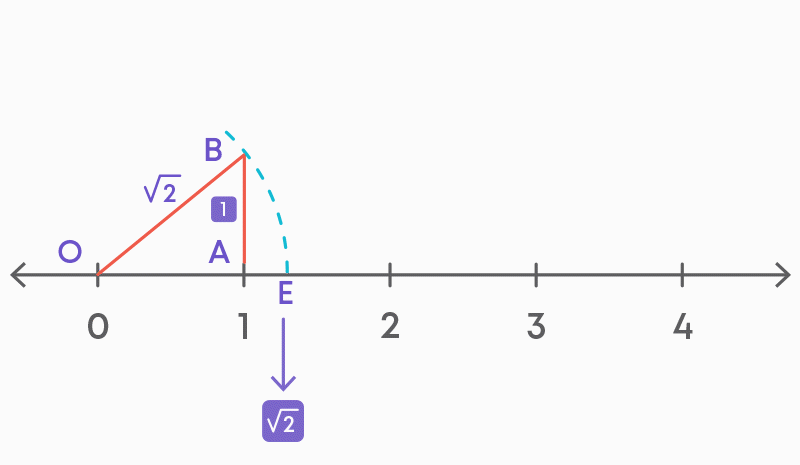Now let us check if irrational numbers satisfy commutative property under addition, subtraction, multiplication, and division.

Irrational numbers are commutative under addition and multiplication. For any two irrational numbers ‘m’ and ‘n’,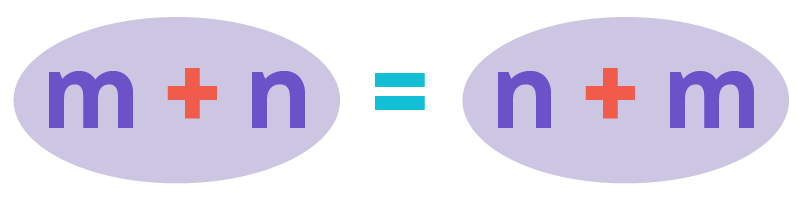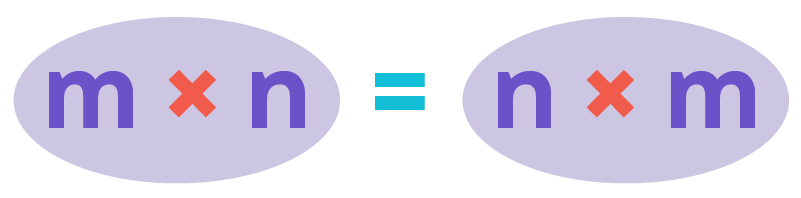Irrational numbers are not commutative under subtraction and division.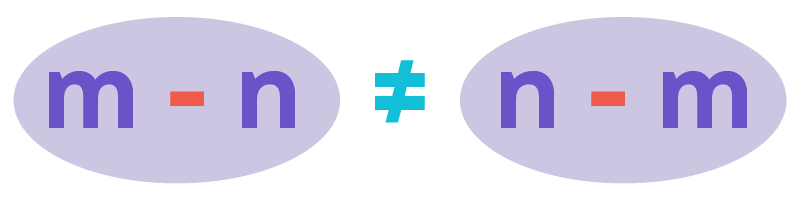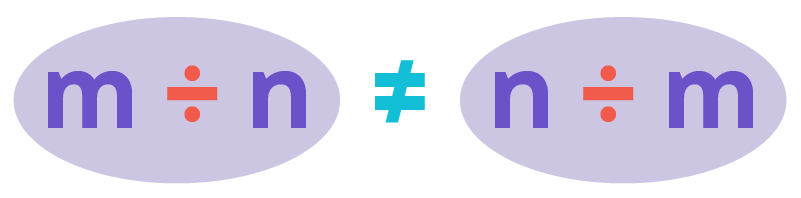Irrational numbers satisfy associative property for addition and multiplication. For any two irrational numbers ‘m’, ‘n’ and o,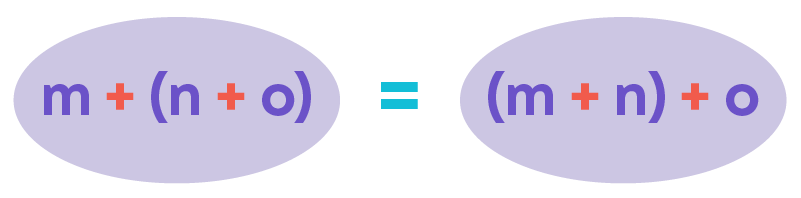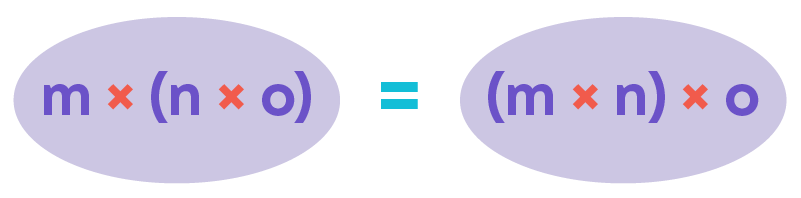Irrational numbers do not satisfy associative property for subtraction and division.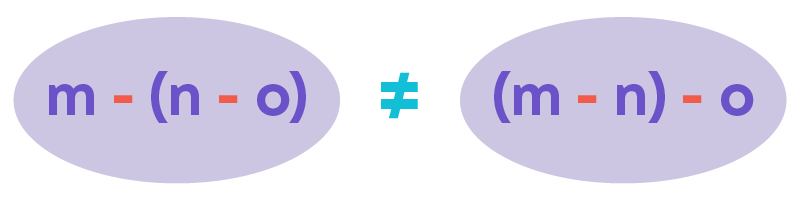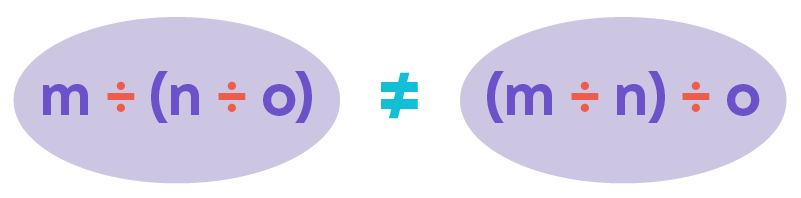Irrational numbers satisfy the distributive property of multiplication over addition. For any two irrational numbers ‘a’, 'b' and 'c':

a × (b + c) = (a × b) + (a × c)

Irrational numbers satisfy the distributive property of multiplication over subtraction. For any two irrational numbers ‘a’, 'b' and 'c':

a × (b - c) = (a × b) - (a × c)

Real Numbers

What can we observe from the given number line?Between any two rational numbers, we can have a rational or an irrational number. The collection of all rational and irrational numbers put together are called real numbers. The number line can also be called as a real number line. Every real number is either rational or irrational. Between any two real numbers, there exist infinitely many real numbers.

All the numbers on the real number line are real numbers. On the number line, each point corresponds to a unique real number and every real number can be represented by a unique point on the real line. Thus, like rational and irrational numbers, there exist infinitely many real numbers between any two real numbers.

Let us now learn about terminating and non-terminating decimals.

The decimal form of 1 2 is 0.5. The decimal form of 3 4 is 0.75. The decimal form of 7 8 is 0.875.

In the above decimal numbers, we can observe that there are finite number of digits in the decimal place or after the decimal point that is the decimal places terminate.

If there are finite number of digits after the decimal points, such decimal numbers are called terminating decimals.Now, observe the decimal form of 1 3 .

1 3 = 0.3333…

Here, the digits in the decimal place do not terminate. Such decimal numbers are called non-terminating decimals.

If the digits in the decimal place are infinite, that is they do not terminate, then such decimals are called non-terminating decimals.

Terminating decimals are decimal numbers in which the digits after the decimal point terminate at a finite number. Example: 0.0625, 0.25, 0.5

The decimal numbers that do not terminate and the digit in the decimal place keeps on repeating are called non-terminating repeating or recurring decimals.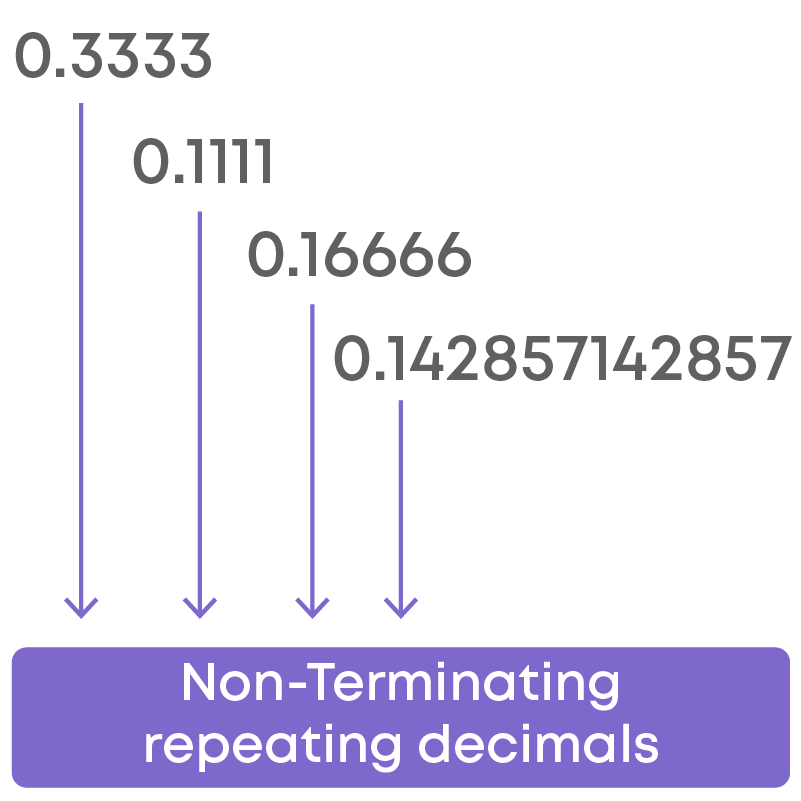Consider the decimal numbers:

1.333333… and 3.121212…

What is the difference between the two decimal numbers? In 1.3333…, only one digit is repeated in the decimal place. In 3.121212…, two digits are repeated in the decimal place.

If a recurring decimal is taken, we will have the same digit or a set of digits periodically repeating in the decimal place. How can such numbers be represented?

We can put a bar on the numbers that repeat periodically. Example: 0.124124124… can be written as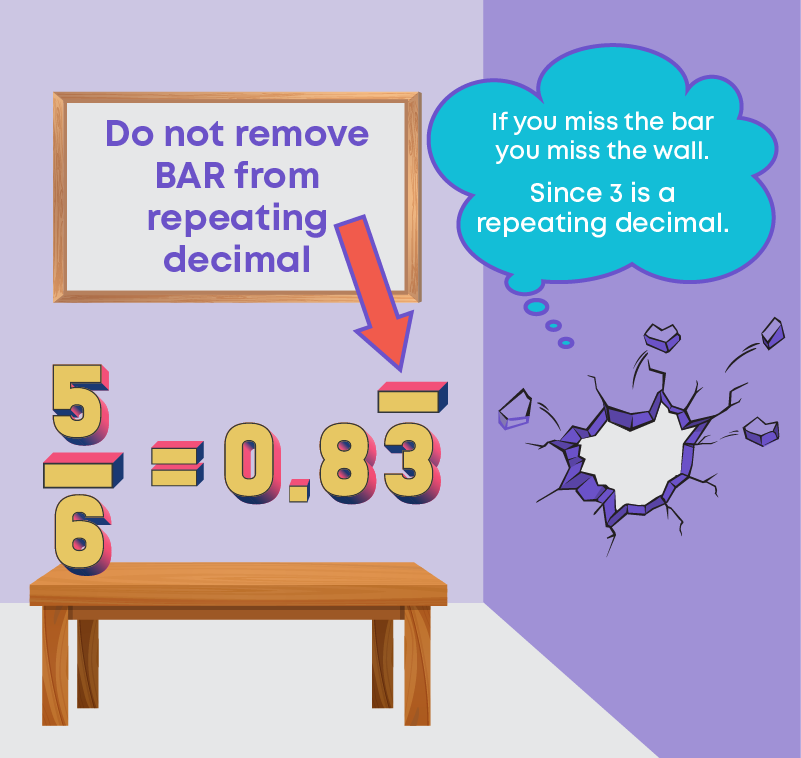A non-terminating decimal is written with the required number of digits in the decimal place and dots are placed at the end. The dots at the end indicate that it is a non-terminating decimal.

Let us learn how to convert a decimal expansion to the form of p q .

Example: convert 0.2500 to the form of p q .

Step 1: Determine the number of digits in the decimal part

Number of decimal parts in 0.2500 is 4.

Step 2: Write the decimal number in rational form

Remove the decimal point from the numerator. Write 1 in the denominator and put as many zeros on the right side of 1 as the number of digits in the decimal part.

0.2500 = 2500 10000

Step 3: Reduce the rational number to simplest form

The common divisor of 2500 and 10000 is 2500.

2500 10000 = 2500÷2500 10000÷2500 = 1 4

So, the number in its simplest form is 1 4 .

Now let us see how to convert recurring decimal numbers in the form of p q .Here, only 1 digit is repeating. Since, we have only one digit repeating, we multiply the equation by 10.

Thus, the rational number is 4 9 .

Similarly, if there are three digits that are repeating successively after the decimal, multiply the equation by 1000.

Terminating decimals can be expressed in the form of p q , where p and q are integers and q ≠ 0. Thus, we can conclude that terminating decimals are rational numbers. Also,

non-terminating recurring decimals can be expressed in the form of p q , where p and q are integers and q ≠ 0. Thus, we can conclude that non-terminating recurring decimals are rational numbers.

Now, let us discuss the steps to find the decimal expansion of the square root numbers given below:

Step 1: Write 2 as a decimal number with some pairs of zeros after the decimal point and pair the digits

In the integral part, we pair the digits from right to left. Here, we have only one digit, so place a bar over the digit 2. In the decimal part, place a bar over each pair of digits starting from the left to right.

2. 00 00 00

Step 2: Consider the digit/s on the integral part of the decimal.

Find the largest number whose square is less than or equal to 2. The number is 1. Take 1 as the divisor and 2 as the dividend. Divide and get the remainder.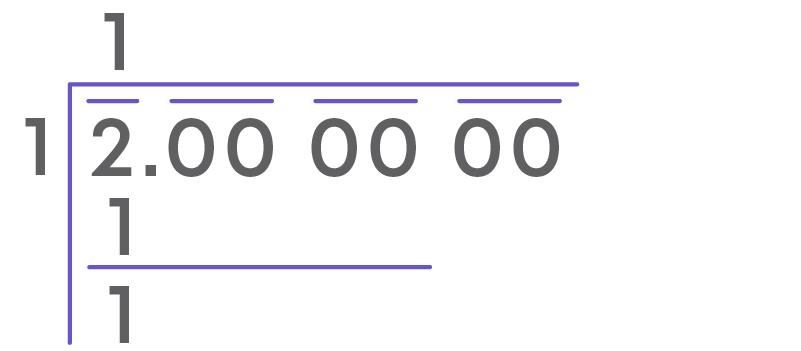Step 3: Bring down the next pair of numbers to the right of the remainder. This new number 100 is the new dividend.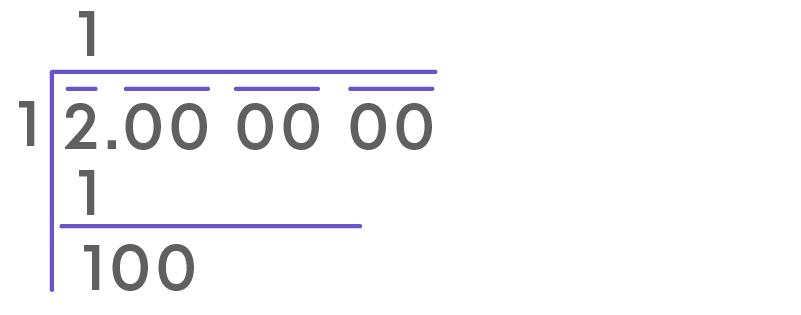Step 4: Add the divisor and quotient (1 + 1 = 2) and put a blank on the right of the number. The blank is for the next digit of the new divisor.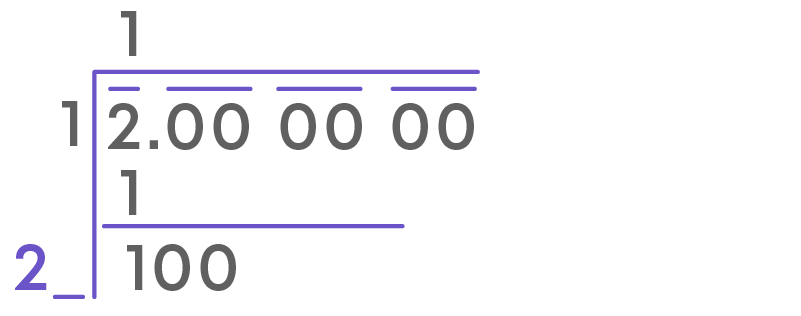Step 5: Find the largest possible digit which will fill the blank and will also be the quotients next digit

Any of the following digit can replace the blank -

21 × 1 = 21

22 × 2 = 44

23 × 3 = 69

24 × 4 = 96

25 × 5 = 125Continue the above process till we get the remainder as 0 or till we get the desired number of digits in the decimal place.

digits in the decimal place. Since irrational numbers are non-terminating and non-recurring decimals, we can divide the numbers up to 3 or 4 decimals.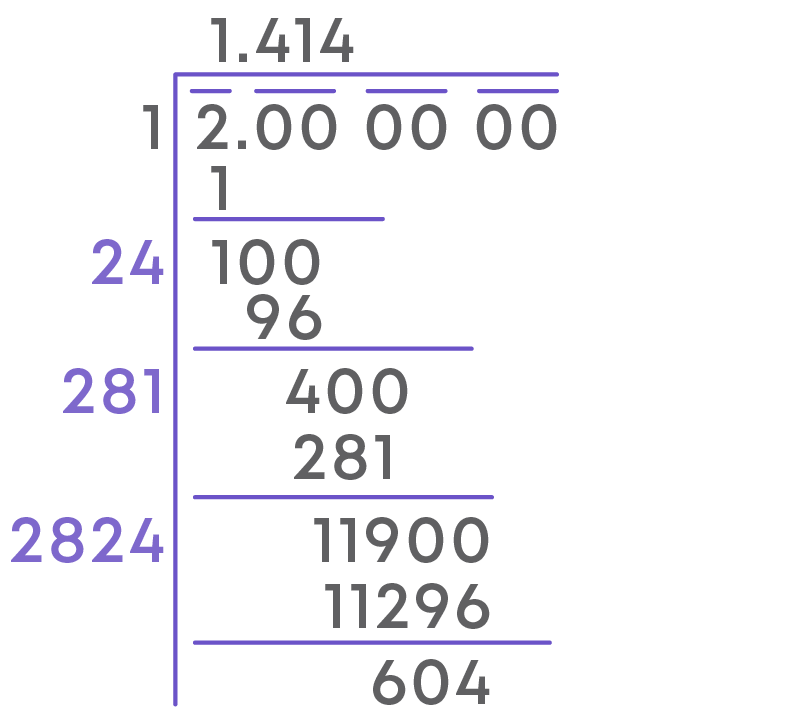By following this process, we can find the decimal expansion of the square root.

The decimal expansion of irrational numbers will be non-terminating non-recurring.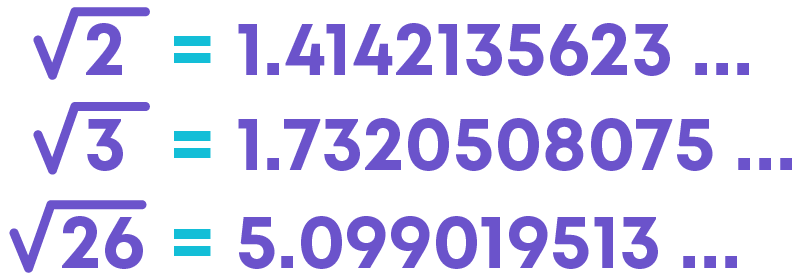Above are examples of irrational numbers. Hence, we can say that all non-terminating non-recurring decimals are irrational numbers.

#### Real Numbers on a Number Line

Rational numbers and irrational numbers can be located on a number line. We know that real numbers are either rational or irrational numbers. Thus, locating a real number is the same as locating a rational or an irrational number.

To represent the square root of a decimal number geometrically on a number line, follow these steps:

Consider the real number √3.5.

Step 1: Draw a line AB = 3.5 units.

Mark the distance 3.5 units from a fixed-point A on a given line to obtain a point B such that AB = 3.5 units.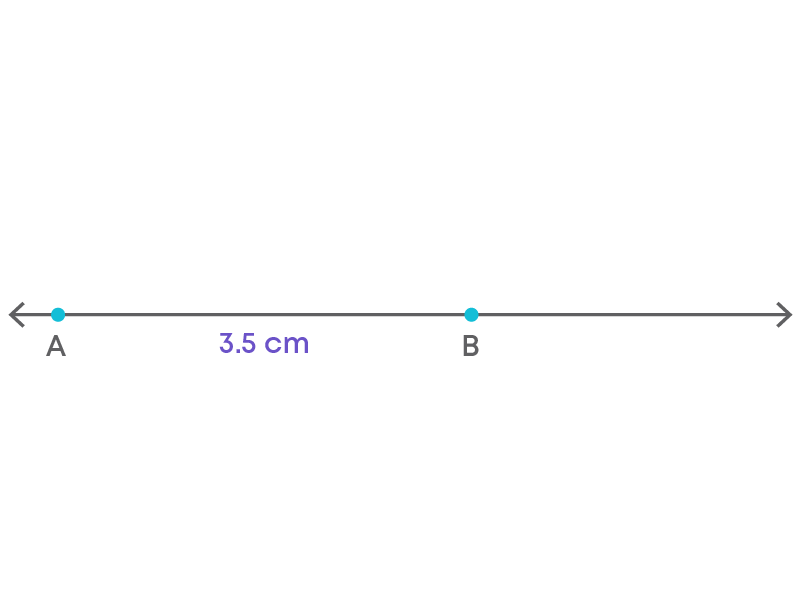Step 2: Extend the line AB by 1 unit.

From B, mark a distance of 1 unit and mark the new point as C. Join BC.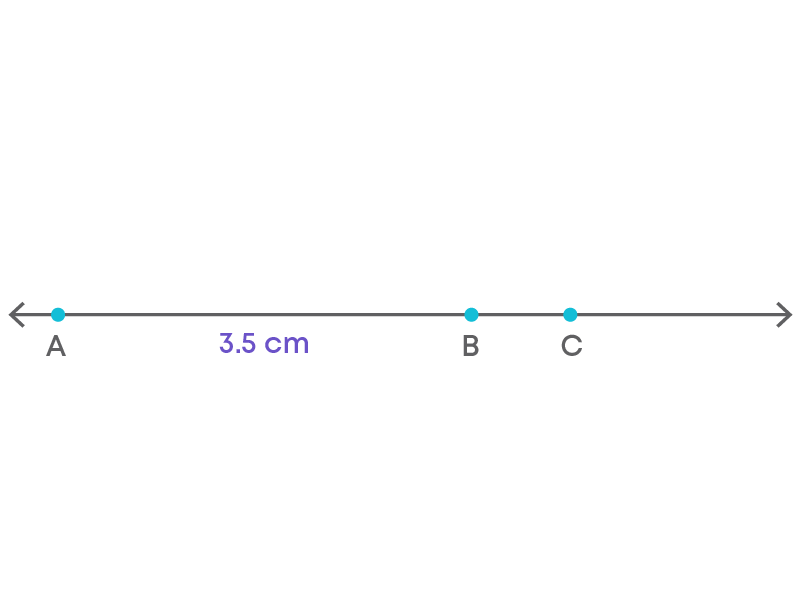Step 3: Find the mid-point of AC and mark that point as O.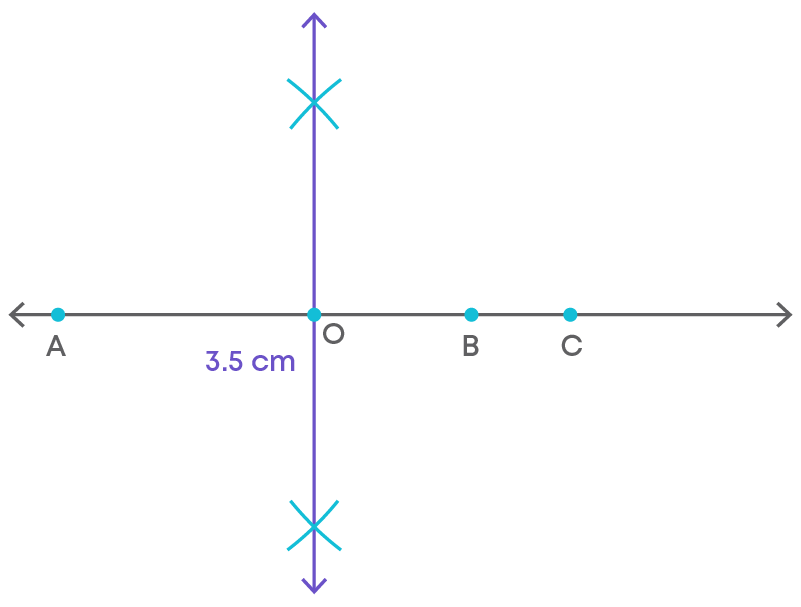Step 4: Draw a semicircle with centre O and radius OC.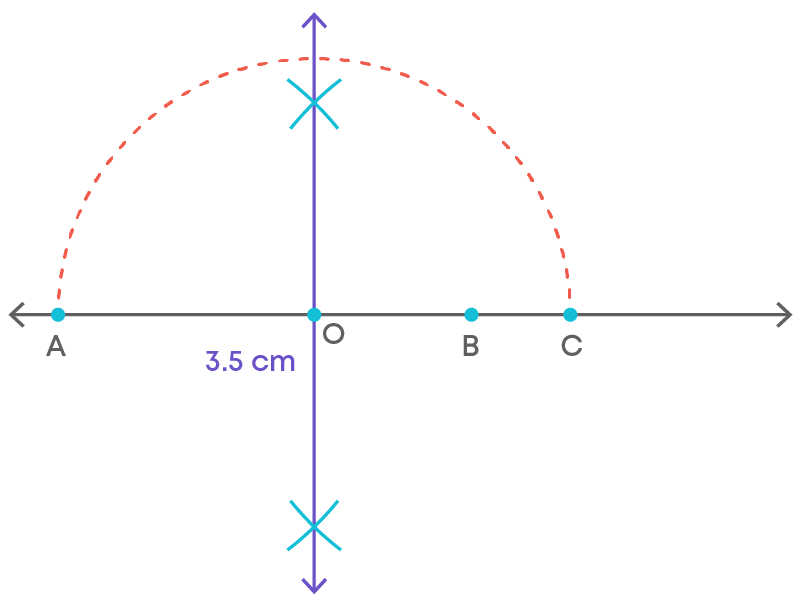Step 5: Draw a line perpendicular to AC passing through B and intersecting the semicircle at D. Draw an arc with centre B and radius BD, which intersects the number line in E.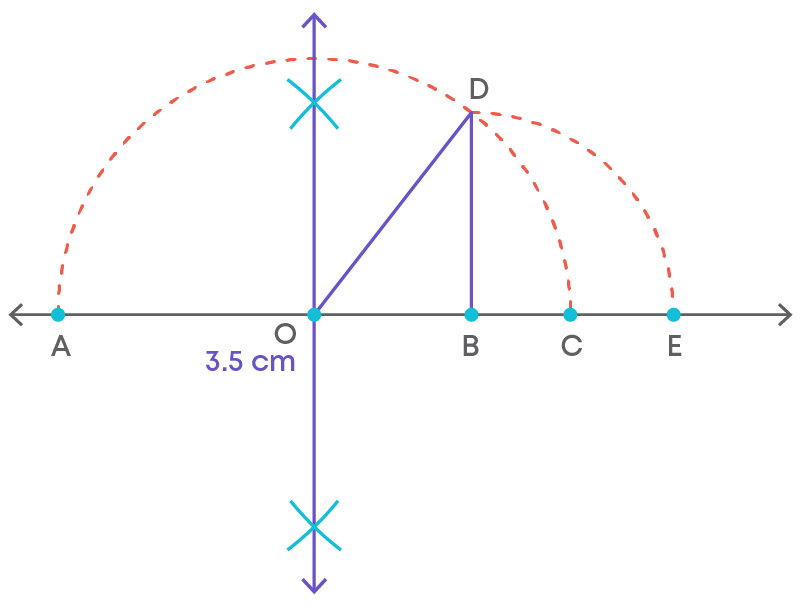The point E represents the real number √3.5.

To determine which real number is represented on the number line:

• Observe the number line.
• Check the length represented by the hypotenuse of the right-angled triangle.
• This length represents the real number (under a square root).

Example: In the figure below, the hypotenuse is √n. Hence, the point C represents the real number √n.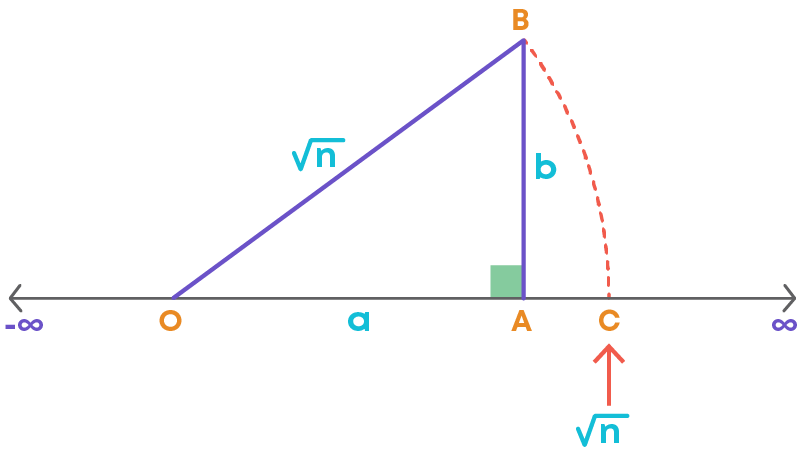The following shows the representation of the square root of a decimal number. In this case, observe the length BD. The point E represents the square root of the length BD.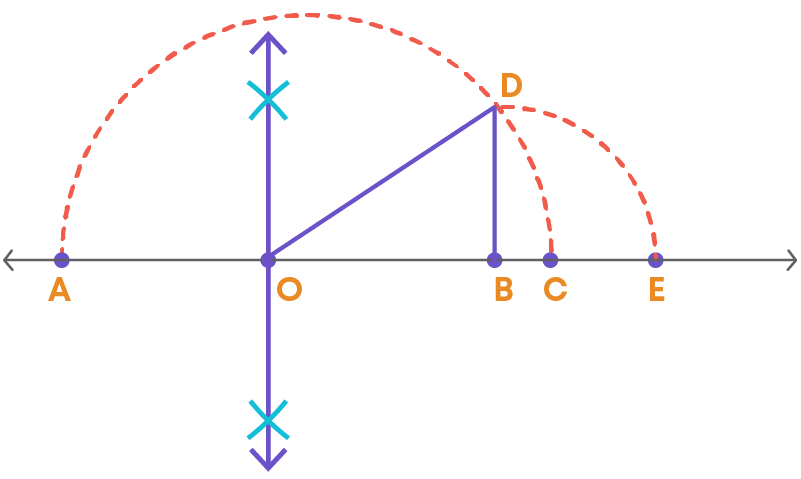Can we represent the decimal expansions of rational and irrationals on a number line? Yes, we can represent the decimal expansion on the number line. So, how do we locate 3.6 on a number line?

The number 3.6 is a rational number, that is a terminating decimal.

Step 1: By observing the number line we find that 3.6 lies between 3 and 4.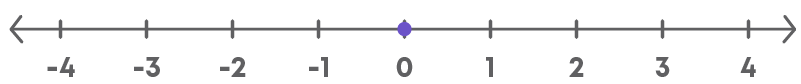Step 2: We divide this into 10 equal parts and mark them as 3.1, 3.2, 3.3, 3.4 so on.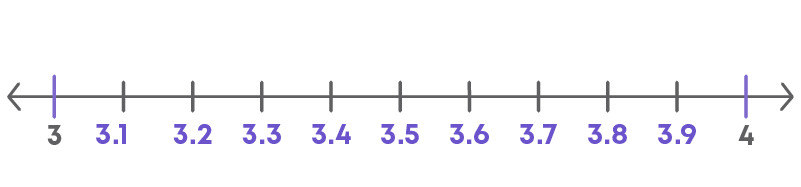Step 3: From 3 we need to move 6 steps forward to locate 3.6.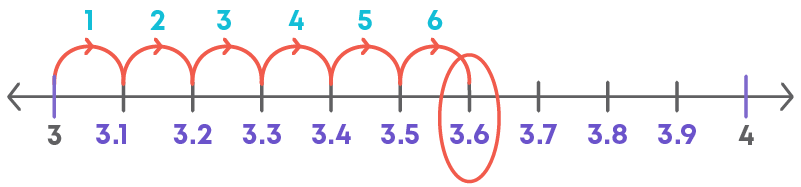Hence, we can locate 3.6 on the number line.

We have discussed, how to represent terminating decimals on a number line. How do we locate a recurring decimal, say 3.6 on the number line?

The number 3.6 is a non-terminating, recurring decimal. So let us locate the decimal for the first four decimal places.

3.6 or 3.6666…. is between the whole numbers 3 and 4.Thus, we divide the number line between 3 and 4 into 10 equal parts.Now, we need to move 6 steps forward between 3 and 4 to locate 3.6.Again, we need to divide the number line into 10 equal parts between 3.6 and 3.7. Now, we need to move 6 steps forward between 3.6 and 3.7 to locate 3.66.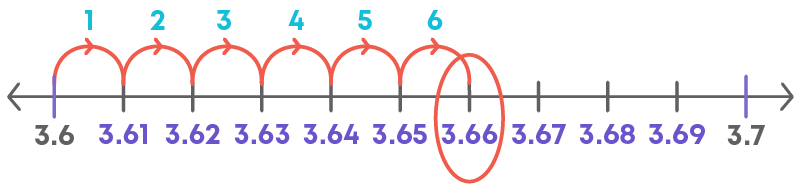Again, we need to divide the number line into 10 equal parts between 3.66 and 3.67. Now, we need to move 6 steps forward between 3.66 and 3.67 to locate 3.666.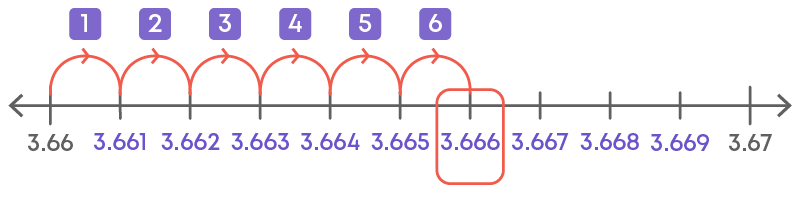Now, we divide the number line between 3.666 and 3.667 into 10 equal parts and locate 3.6666.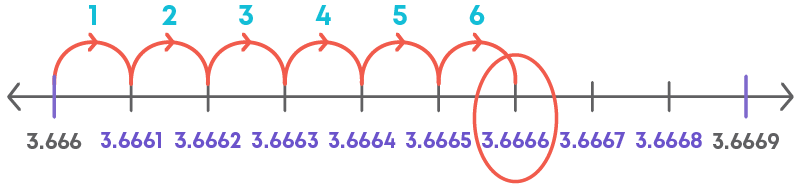Hence, we can locate 3.6666 on the number line. When we observe each step, we magnify the number line successively to find the required decimal.

Thus, finding the decimal on a number line using magnification is called the successive magnification method.

The process of visualisation of numbers on the number line by successively dividing the number line is called the successive magnification method.

Let us understand the successive magnification method by an example.

Represent 7.7 on a real number line.

Step 1: The number 7.7 lies between 7 and 8.

This means that we need to divide the line segment between 7 and 8 into 10 equal parts. That is, we magnify the number line between 7 and 8.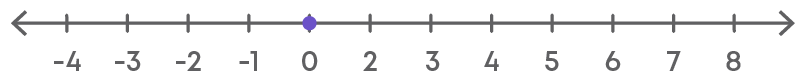Step 2: On dividing the number line between 7 and 8 into 10 equal parts we get 7.1, 7.2, 7.3… and so on.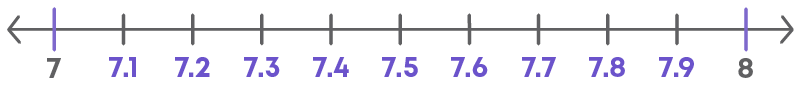Step 3: 7.7 has 7 units (smaller divisions) from 7. Locate and mark the point as P.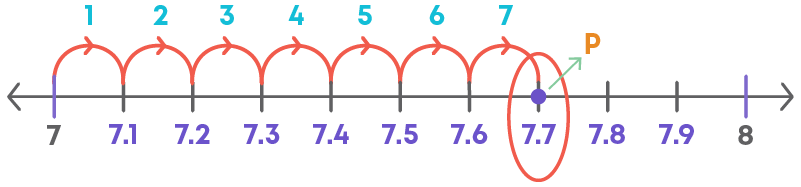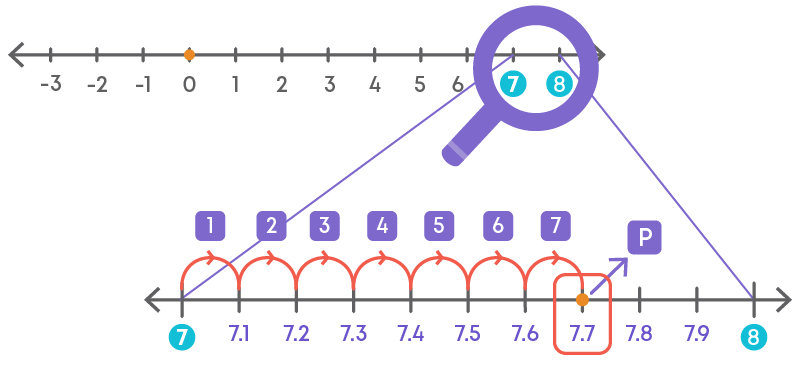Clearly, the point P on the number line represents 7.7.

To locate terminating or non-terminating decimals, we divide the line segment by successive magnification of the number line.

Example: To locate 2.8784, we successively divide the line segment between 2 and 3, then between 2.8 and 2.9, then between 2.87 and 2.88, lastly between 2.878 and 2.879.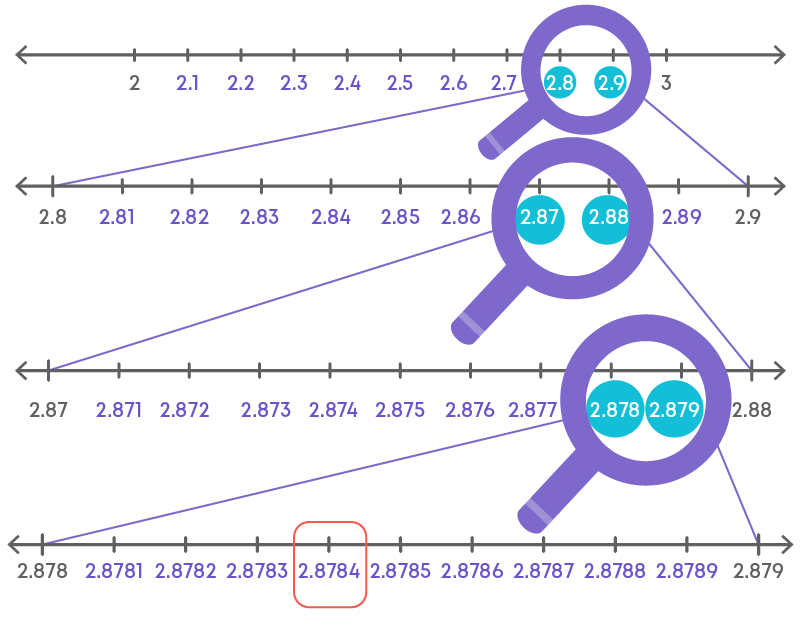#### Operations on Real Numbers

Can we add a rational and an irrational number? Yes, we can add rational and irrational numbers.

Find the sum of the rational 2 8 and irrational √7.

2 8 + √7 cannot be simplified further. Thus, the sum of 2 8 and √7 is 2 8 + √7.

From the previous examples if a rational and irrational number is added, what is the result obtained, rational or irrational? Irrational. When a rational and irrational number are added the result obtained is an irrational number.

Can we subtract a rational and an irrational number? Yes, we can subtract a rational and irrational number. Let us discuss an example and understand more about the difference between a rational and an irrational number.

Consider a rational number 3 and an irrational number √5. Let us find their difference. Subtracting √5 from 3 is 3 – √5.

3 – √5 cannot be simplified further. Thus, the difference of √5 from 3 is 3 – √5.

From the previous examples if a rational and an irrational number is subtracted, what is the result obtained, rational or irrational? Irrational. When a rational and an irrational number is subtracted then the result obtained is an irrational number.

While adding two irrationals with the same irrational coefficient, we add their rational co-efficient and keep the irrational coefficient as it is. Example:

11√3 + 2√3 = (11 + 2)√3 = 13√3

When we add two real numbers, the sum is also a real number.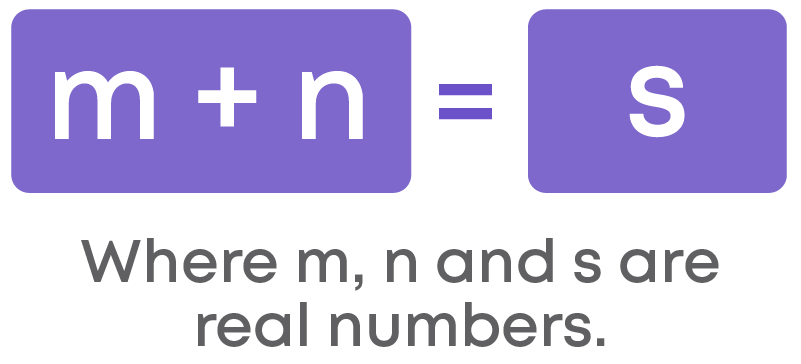The difference of a rational and an irrational number can be a rational or an irrational number. Thus, the difference of two real numbers is a real number.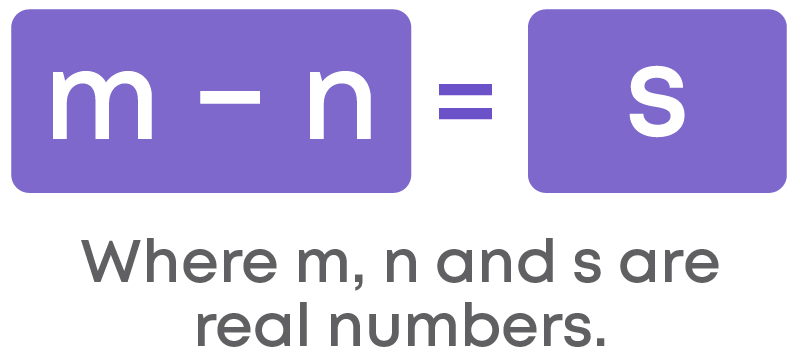Let us consider the difference between two real numbers 2√3 and 5√3.

In these numbers, we find that the irrational coefficients are same but the rational coefficients are different. To subtract them, we subtract the rational coefficients and keep the irrational coefficient as it is.

2√3 - 5√3 = (2 – 5)√3 = -3√3

When a rational and an irrational number is multiplied, the result obtained is an irrational number.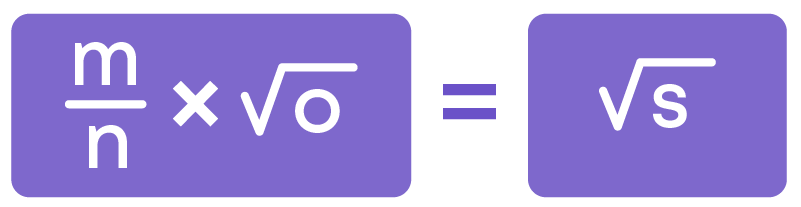The product of two irrational numbers is also irrational.

Thus, when two real numbers are multiplied the obtained result is a real number.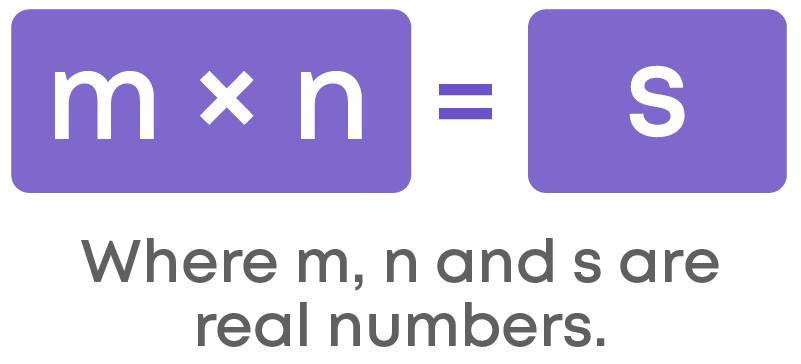Let us multiply two real numbers 4 and 3√2.

Here, we multiply the rational coefficients. So, we have 4 × 3√2

= 12√2

When a rational and an irrational number is divided the result obtained is an irrational number. We can also say that when we divide two non-zero real numbers, the result is a real number.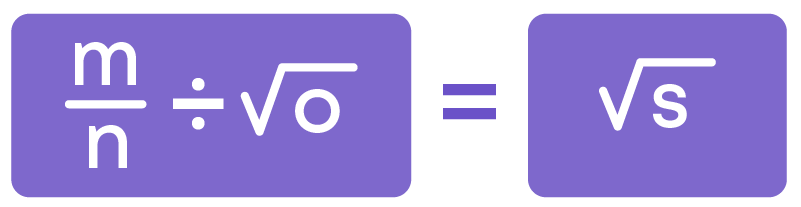Where m, n, o, and s are real numbers.

The quotient of two irrational numbers will be either rational or irrational.

Let us divide √24 and 3√6.

√24 3√6 = √4×6 3√6 = 2√6 3√6 = 2 3

To simplify expressions with real numbers, remember:

• To check the operations involved.
• Use appropriate identities to simplify the terms in the expression.
• Combine like terms to find the result.

Real numbers are closed under addition, subtraction, and multiplication but not under division.

This means:

• Sum of two real numbers is a real number.
• Difference of two real numbers is a real number.
• Product of two real numbers is a real number.
• Quotient of two non-zero real numbers may not be a real number.

Real numbers satisfy commutative property under addition and multiplication. Real numbers do not satisfy commutative property under subtraction and division.

Examples: ’a’ and ‘b’ are two real numbers where a = 2 + √5 and b = 2 - √5

a + b = 2 + √5 + 2 - √5 = 4

b + a = 2 - √5 + 2 + √5 = 4

a + b = b + a

a b = 2 + √5 2 - √5

b a = 2- √5 2+ √5

a b a b

Real numbers satisfy associative property under addition and multiplication, but not under subtraction and division.

For example, let a = 2 + √5, b = 2 - √5, c = 2√5.

a × (b × c)

2 + √5 × ((2 - √5 × 2√5))

(2 + √5) × (4√5 – 2 × 5)

(2 + √5)( 4√5 – 10)

= 8√5 – 20 + 20 – 10√5

= -2√5

(a × b) × c

((2 + √5) × (2 - √5)) × 2√5

= (4 – 2√5 + 2√5 – √5 × √5) × 2√5

= (4 – 5) × 2√5

= -2√5

So, a × (b × c) = (a × b) × c

The additive identity of any real number is zero. Example: √5 + 0 = √5

Consider the real number 5 + √5.

(5 + √5) + 0 = 5 + √5

1 is called the multiplicative identity of real numbers. Example: (√7 + 1) × 1 = √7 + 1

The sum of a real number and its negative is 0. This means that for every real number there exists its additive inverse such that their sum is 0.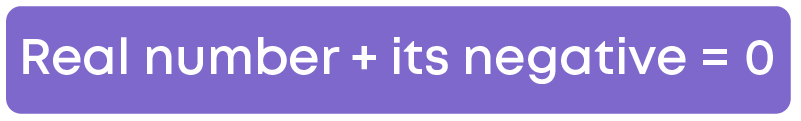Product of a real number and its reciprocal is 1.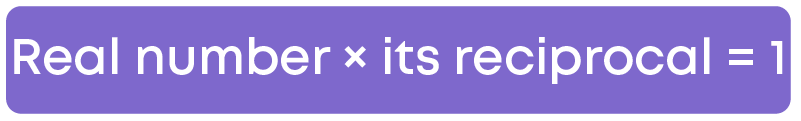Hence, the multiplicative inverse of any real number is its reciprocal. Example: the reciprocal of
2 + √3 is 1 2+√3 . Their product: 2 + √3 × 1 2+√3 = 1.

Real numbers also satisfy distributive property under multiplication over addition.

(a × b) + c = (a × b) + (a × c) where ‘a’, ‘b’ and ‘c’ are real numbers.

Rationalisation

If the denominator of a number is irrational, then the process of converting the given number into its equivalent expression whose denominator is a rational number is called rationalisation.

For this, we multiply the numerator and the denominator by the rationalising factor. If the product of two irrational numbers is rational then each one is called the rationalising factor of the other. Example: 7 √11

On multiplying the numerator and denominator of the given number by √11, we get:

7 √11 x √11 √11 = 7√11 √11

Here the rationalising factor is √11.

Consider another irrational number 2 √3 . Let us rationalise it.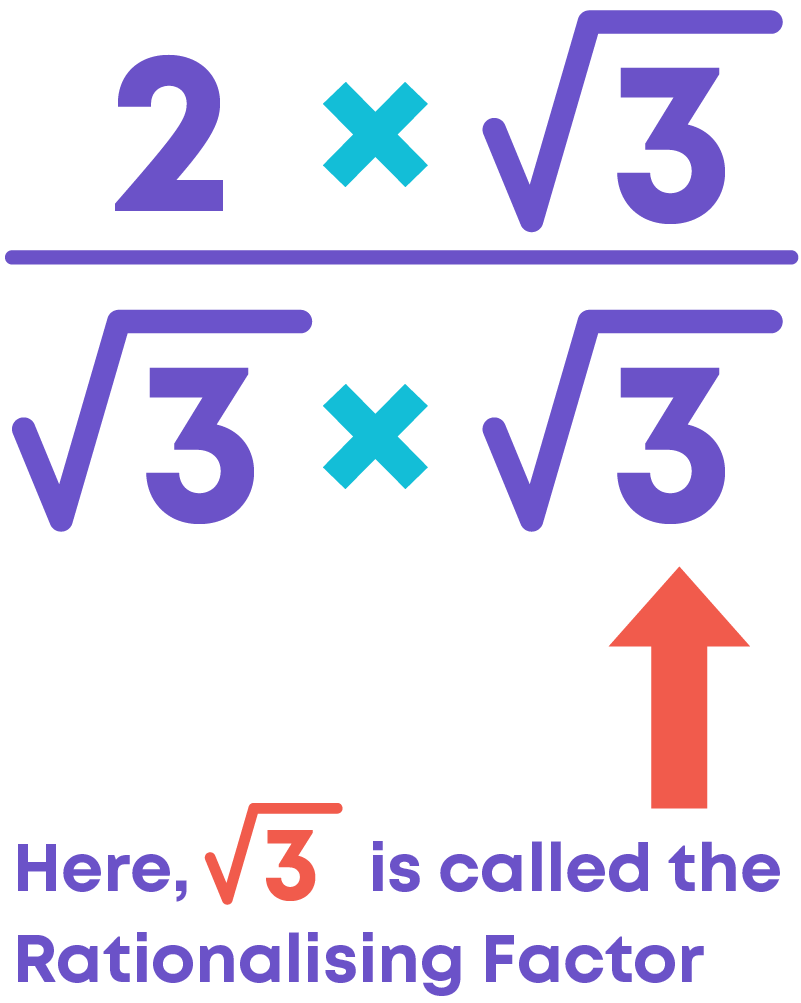Let us learn about the rationalising factor or conjugate of a real number.

The product of (3 + √2)(3 – √2) = 7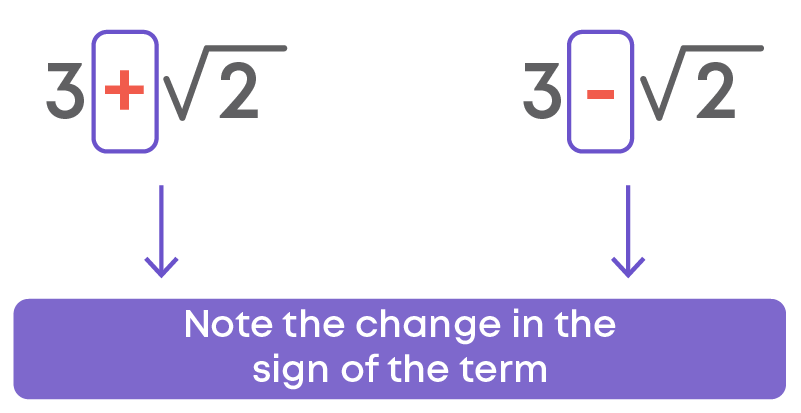The sign of the term is opposite. When we multiply the two irrational numbers, we get a rational number. Can we say that each is a rationalising factor of the other? Yes.

Here, 3 – √2 is called the rationalising factor or the conjugate of 3 + √2.

If the product of two irrational numbers is a rational number, then each irrational number is a rationalising factor of each other.

To find the conjugate of a binomial, we just change the sign between the terms.

#### Laws of Exponents for Real Numbers

When any number is multiplied by itself repeatedly, the multiplication can be represented in the form of an exponent.

If ‘a’ is any integer, then

1) a × a × a

Here, ‘a’ is multiplied by itself 3 times. This can be written in the exponent form as a3

2) If ‘a’ is multiplied by itself ‘m’ times, then the multiplication can be written in the exponent form as am

a × a × a × a………..’m’ times = am

Let us recall the laws of exponents when the powers are integral. For a non-zero integer ‘a’ and whole numbers ‘m’ and ‘n’, we have

Law 1: Product Rule

am × an = a m+n

Law 2: Quotient Rule

am ÷ an = am-n

Law 3: Power of a Power Rule

(am)n = amn

Law 4: Power of a Product Rule

am × bm = (ab)m

Law 5: Power of a Fraction Rule

am bm = ( a b ) m

Law 6: Zero Power

a0 = 1

Law 7: Negative Power

a-1 = 1 a

Let us practice applying the laws of exponents.

Consider two real numbers say 5 and 7.

1) What is the value of (5 × 7)2?

According to the law of exponents, we know that (ab)n = anbn

Thus, (5 × 7)2 = 52 × 72 = 25 × 49 = 1225

2) What is the value of ( 5 7 )2?

According to the law of exponents, we know that ( a b )n = an bn , b ≠ 0

Thus, ( 5 7 )2 = 52 72 = 25 49

(92)2 and ( 1 7 )2 are real numbers whose powers are integrals. Can the powers be rational numbers? Let us see:

Consider the square root of 4. It is written as √4.

√4 = 2………………(1)

Now, √22 = 2

√2 = 2 1 2

2 can be expressed as 2 (2) 1 2

2(2) 1 2 = (4) 1 2 = 2………………………….(2)

From (1) and (2), we can see that:

√4 = 2(2) 1 2 = (4) 1 2 = 2

So, we can say, √4 = (4) 1 2

From this example, we can see that for any positive real number ‘a’,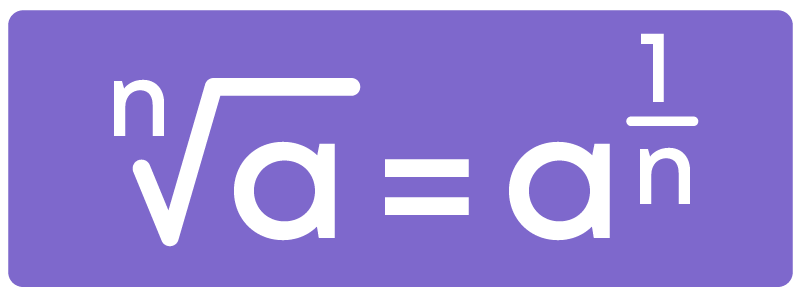From this, we can see that real numbers can have a rational exponent.

Let us explore the multiplication rule of real numbers with rational powers:

Consider 2 1 2 × 2 1 3

Which law can we apply to simplify this? We know that for any integral powers, the product rule of exponents states am × an = am+n

Let us apply the same for exponents with rational powers:

2 1 2 × 2 1 3 = 2 ( 1 2 + 1 3 )

= 2( 3+2 6 )

= 2 ( 5 6 )

Let us explore the division rule of real number with rational powers:

Consider 2 1 2 2 1 3

Let us simplify this using the law of division rule of exponents, am ÷ an = am-n

2 1 2 ÷ 2 1 3 = = 2( 1 2 - 1 3 )

= 2( 3-2 6 )

= 2( 1 6 )

How can we represent a negative rational power as a positive rational power? Consider the

exponent: 3- 3 2

According to the law of exponents, a-1 = 1 a

Let us apply this law,

3 - 3 2 = 1 3 3 2

Let us learn simplification using power of power rule of exponents.

(25) ( 1 4 ) 1 5 is a real number. Can we simplify using the law of exponents? Yes. Which law can we apply for this? We can use the power of power rule of exponents which states,

(am)n = amn

Thus, (25) ( 1 4 ) 1 5 = 25 1 4 x 1 5 = 25 1 20

Let us learn simplification using power of product rule of exponents.

(2 ×5) 3 4 is a real number. Let us simplify this using the law: am × bm = (ab)m

(2 ×5) 3 4 = 2 3 4 5 3 4

We can also simplify real numbers using the power of 'n'th root of a real number.

Examples: ∛5 = 5 1 3 , (∛7)2 = (7 1 3 )2 = 7 2 3

#### Common Errors

The following are topics in which students make common mistakes when dealing with number systems:

• 1. -√7 is not the same as √-7
• 2. Not all square root numbers are irrational numbers
• 3. If we add, subtract, multiply or divide two irrational numbers, the result need not be always an irrational number.
• 4. In locating a square root of a number on a number line, the first step is to choose two numbers such that the sum of the squares of two numbers is equal to given number (without root), and the chosen number need not always be a natural number.
• 5. When rationalizing the denominator, the conjugate of the denominator is always in the opposite sign.

#### -√7 Is Not The Same As √-7

-√7 is an irrational number with negative sign. √-7 is an imaginary number.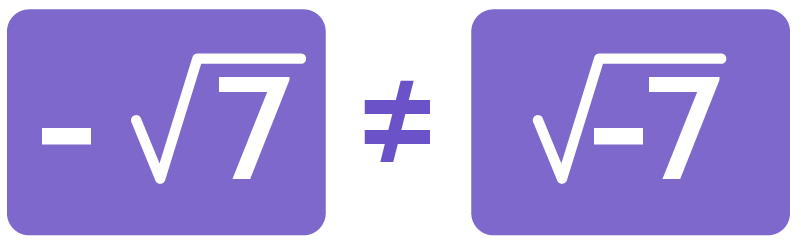#### Not All Square Root Numbers Are Irrational Numbers

Consider √25 and √5.

√25 = √5×5 = √52 = 5, which is a rational number. √5 is an irrational number, since it cannot be expressed in p q form.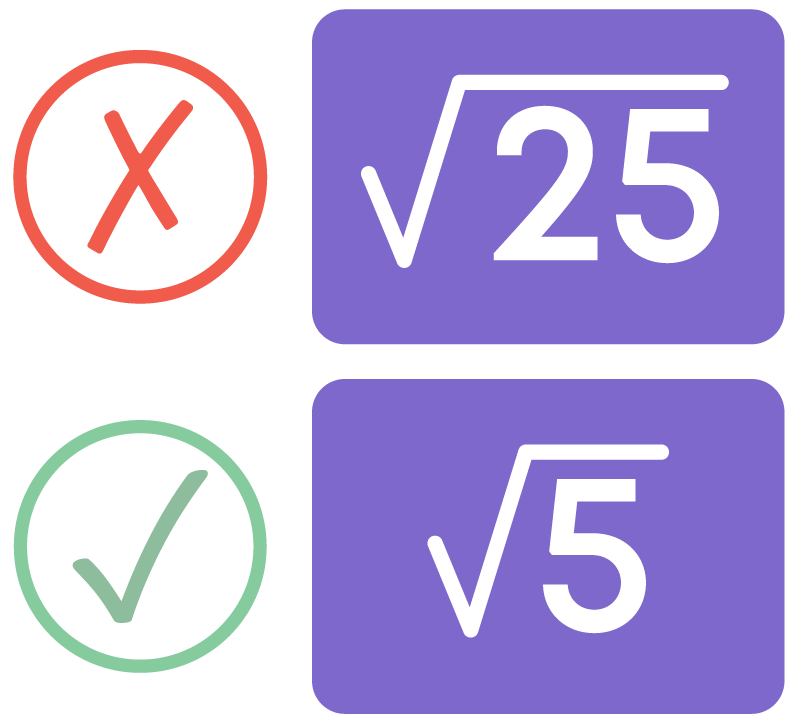Square root numbers are said to be irrational if the number inside the square root is not a perfect square.

#### If We Add, Subtract, Multiply Or Divide Two Irrational Numbers, The Result Need Not Be Always An Irrational Number.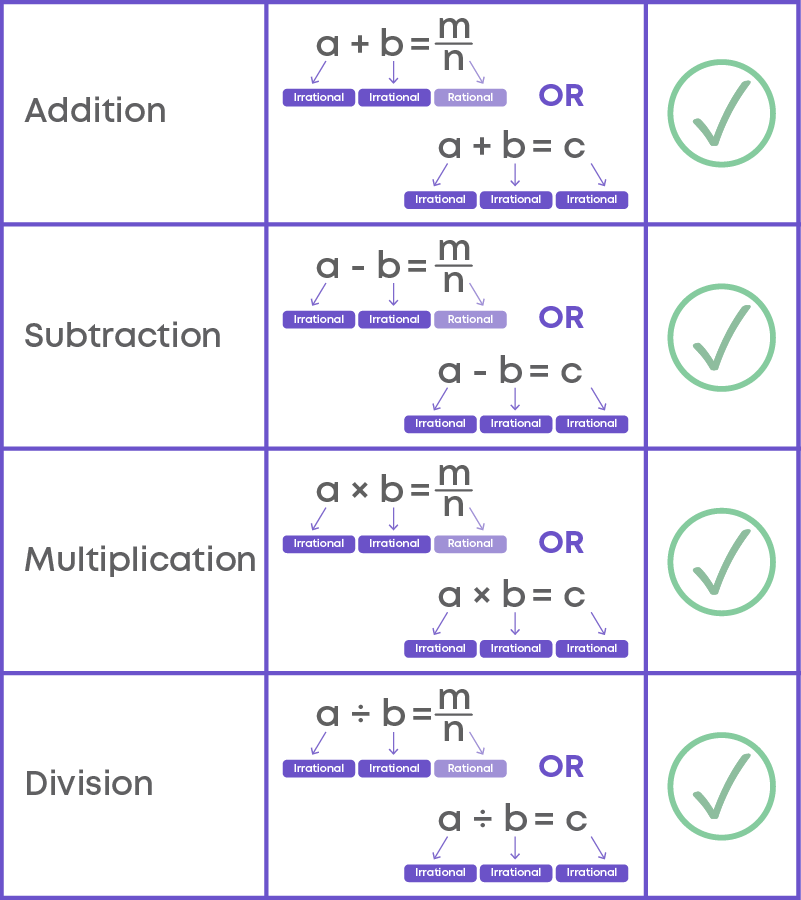#### In Locating A Square Root Of A Number On A Number Line, The First Step Is To Choose Two Numbers Such That The Sum Of The Squares Of Two Numbers Is Equal To Given Number (Without Root), And The Chosen Number Need Not Always Be A Natural Number.

Consider √7, to locate √7 on a number line, the first step is to choose two numbers such that the sum of the squares of two numbers is equal to given number (without root).

7 = (√6)2 + (1)2

Here, √6 is an irrational number. The chosen number can be both,#### When Rationalizing The Denominator, The Conjugate Of The Denominator Is Always In The Opposite Sign.

For example, consider the irrational number √5 (5+√5) .

Denominator of the given number is 5+√5. Conjugate of 5+√5 is 5-√5 (same terms with opposite sign).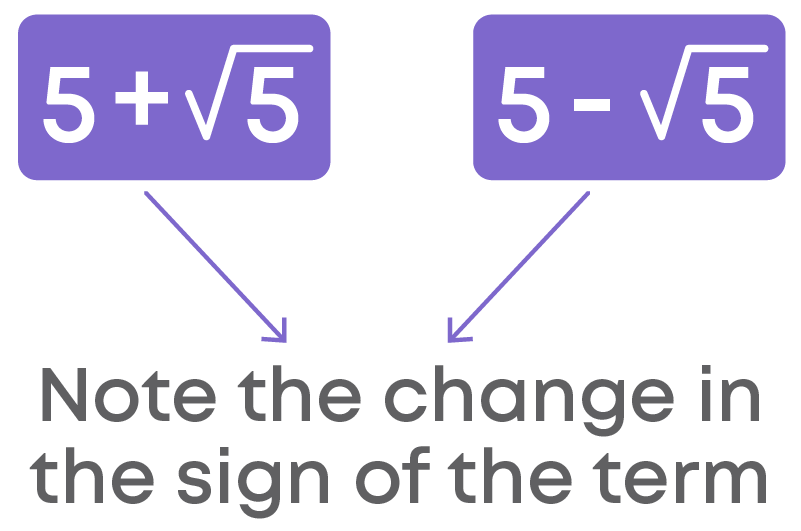#### Conclusion

Can you solve this riddle now that you know about number systems?

Choose the rational number between 11250 625 and 12500 625 among the following options:

(a) 19

(b) 10250 625

(c) 10000 625

(d) 9500 625#### Arpana

Author

Arpana is an education specialist with years of teaching Math and developing content. She previously worked as a freelance content developer, developing lesson plans for a reputed publisher of text books and content for various educational companies. She is an enthusiastic teacher who has taught students from India, the UK, and New Zealand.